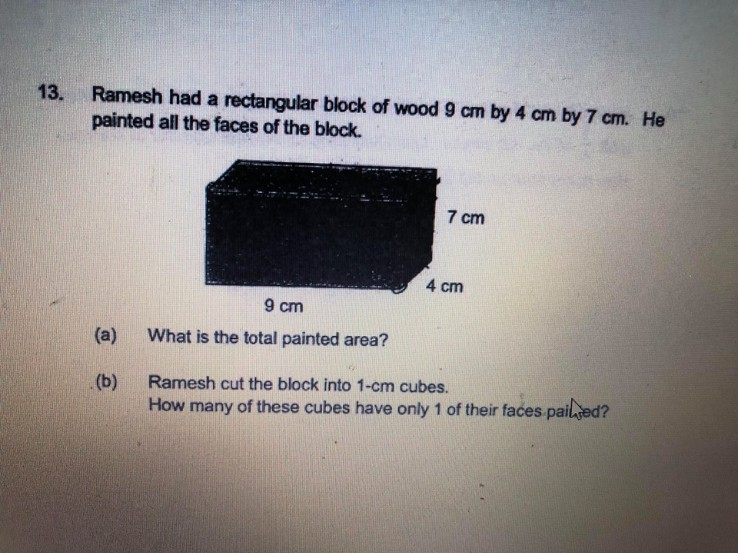# Questionhelp pls

a> Total painted area = surface of the 6 sides

9 x 4 x 2 = 72 cm2
7 x 4 x 2 = 56 cm2
7 x 9 x 2 = 126 cm2

Total area = 72 + 56 + 126 = 254 cm2

b> The 1-cm cubes that have only 1 of their faces painted are those on the 6 sides excluding the edges.

That is to say for the side of 9 x 4
There will be 36 1-cm cubes. The one with only 1 side painted are

(9-1-1) x (4-1-1) = 7 x 2 = 14

Apply the theory into all six sides:

(9-2) x (4-2) x 2 = 28
(7-2) x (4-2) x 2 = 20
(7-2) x (9-2) x 2 = 70

Total number of 1-cm cubes that have only 1 of their faces painted = 28 + 20 + 70 = 118